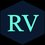# Groups..

Groups is a very important sub-topic in COMBINATORICS which has a lot of significance in study of Combinations. To know what basically a group is, let us take a simple example. Suppose we have 4 apples and we are asked to find the number of ways in which we can select two apples. We all know that it is 4C2 = 6. Let us denote each apple by A,B,C and D . So, all possible combinations are (A,B), (A,C), (A,D), (B,C), (B,D) and (C,D) .

When we chose (A,B) we were left with (C,D). When we chose (A,C) we were left with (B,D). When we chose (A,D) we were left with (B,C). So just by taking 3 combinations , we got all the 6 combinations. Let us put our any one selection of apples in box B1 and the remaining apples in box B2. Now, a group is a set which contains both B1 and B2. So, total number of combinations we can form is 6 but the total number of groups is just 3.

A single group can contain 'n' number of elements where 'n' is a natural number. In the above case , there were two elements , B1 and B2.

Now, given below are some basic formulas for groups which will help in solving many groups problems....

1. The number of ways in which , (m+n) items be divided into two unequal groups containing 'm' and 'n' items is

$\left( \begin{matrix} m+n \\ m \end{matrix} \right) =\left( \begin{matrix} m+n \\ n \end{matrix} \right) =\frac { (m+n)! }{ m!n! }$

2.The number of ways in which 'mn' different items can be divided equally into 'm' groups each containing 'n' items in which order of groups is

a. Not Important

$\frac { (mn)! }{ { n! }^{ m }m! }$

b.Important

$\frac { (mn)! }{ { n! }^{ m } }$

3.The number of ways in which (m+n+p) things can be divided into 3 different groups containing (m),(n) and (p) things is

$\frac { (m+n+p)! }{ m!n!p! }$

4.The number of ways in which 'n' different things can be arranged into 'r' different groups is

$n!\left( \begin{matrix} n-1 \\ r-1 \end{matrix} \right)$

SORRY for any mistake in this note. Actually this is my first note And I insist to mention the mistakes in the comment section so that I can correct it. Please do Like if you think its good.Note by Vighnesh Raut
6 years, 12 months ago

This discussion board is a place to discuss our Daily Challenges and the math and science related to those challenges. Explanations are more than just a solution — they should explain the steps and thinking strategies that you used to obtain the solution. Comments should further the discussion of math and science.

When posting on Brilliant:

• Use the emojis to react to an explanation, whether you're congratulating a job well done , or just really confused .
• Ask specific questions about the challenge or the steps in somebody's explanation. Well-posed questions can add a lot to the discussion, but posting "I don't understand!" doesn't help anyone.
• Try to contribute something new to the discussion, whether it is an extension, generalization or other idea related to the challenge.
• Stay on topic — we're all here to learn more about math and science, not to hear about your favorite get-rich-quick scheme or current world events.

MarkdownAppears as
*italics* or _italics_ italics
**bold** or __bold__ bold
- bulleted- list
• bulleted
• list
1. numbered2. list
1. numbered
2. list
Note: you must add a full line of space before and after lists for them to show up correctly
paragraph 1paragraph 2

paragraph 1

paragraph 2

[example link](https://brilliant.org)example link
> This is a quote
This is a quote
    # I indented these lines
# 4 spaces, and now they show
# up as a code block.

print "hello world"
# I indented these lines
# 4 spaces, and now they show
# up as a code block.

print "hello world"
MathAppears as
Remember to wrap math in $$ ... $$ or $ ... $ to ensure proper formatting.
2 \times 3 $2 \times 3$
2^{34} $2^{34}$
a_{i-1} $a_{i-1}$
\frac{2}{3} $\frac{2}{3}$
\sqrt{2} $\sqrt{2}$
\sum_{i=1}^3 $\sum_{i=1}^3$
\sin \theta $\sin \theta$
\boxed{123} $\boxed{123}$

## Comments

Sort by:

Top Newest

Nice note ...................

- 6 years, 9 months ago

Log in to reply

×

Problem Loading...

Note Loading...

Set Loading...